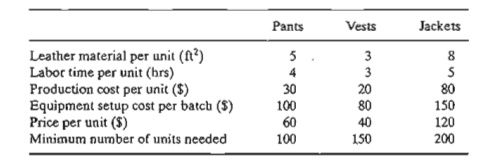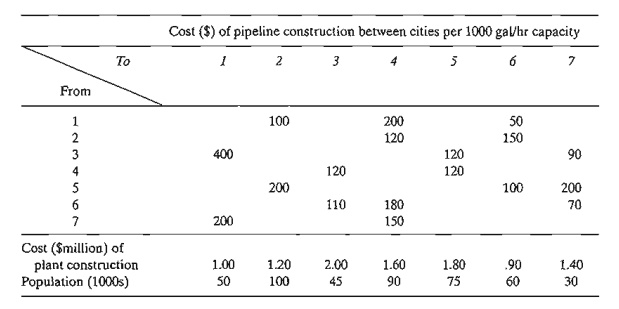Home | | Resource Management Techniques | Fixed Charge Problem- Integer Linear Programming Illustrative Applications

# Fixed Charge Problem- Integer Linear Programming Illustrative Applications

The fixed-charge problem deals with situations in which the economic activity incurs two types of costs: an initial "flat" fee that must be incurred to start the activity and a variable cost that is directly proportional to the level of the activity.

Fixed-Charge Problem

The fixed-charge problem deals with situations in which the economic activity incurs two types of costs: an initial "flat" fee that must be incurred to start the activity and a variable cost that is directly proportional to the level of the activity. For example, the initial tooling of a machine prior to starting production incurs a fixed setup cost regardless of how many units are manufactured. Once the setup is done, the cost of labor and ma-terial is proportional to the amount produced. Given that F is the fixed charge, e is the variable unit cost, and x is the level of production, the cost function is expressed asThe function C(x) is intractable analytically because it involves a discontinuity at x = 0. The next example shows how binary variables are used to remove this intractability.

Example 9.1-3          (Choosing a Telephone Company)

I have been approached by three telephone companies to subscribe to their long distance service in the United States. MaBell will charge a flat \$16 per month plus \$.25 a minute. PaBell will charge \$25 a month but will reduce the per-minute cost to \$.21. As for BabyBell, the flat monthly charge is \$18, and the cost per minute is \$.22. I usually make an average of 200 minutes of long-distance calls a month. Assuming that I do not pay the flat monthly fee unless I make calls and that I can apportion my calls among all three companies as I please, how should I use the three companies to minimize my monthly telephone bill?

This problem can be solved readily without ILP. Nevertheless, it is instructive to formulate it as an integer program.

Define

X1 = MaBelllong-distance minutes per month

x2= PaBelllong-distance minutes per month

x3 BabyBelllong-distance minutes per month

yl  = 1 if x1  > 0 and 0 if x1  = 0

Y2 = 1 if x2  > 0 and 0 if x2  = 0

y3 = 1 if x3  > 0 and 0 if x3  = 0

We can ensure that yj will equal 1 if xj is positive by using the constraintThe value of M should be selected sufficiently large so as not restrict to the variable xj artificially. Because I make about 200 minutes of calls a month, then xj 200 for all j. and it is safe to select M = 200.

The complete model isThe formulation shows that the jth monthly flat fee will be part of the objective function z only if yj = 1, which can happen only if xj > 0 (per the last three constraints of the model). If xj = 0 at the optimum, then the minimization of z, together with the fact that the objective coefficient of yj is strictly positive. will force yj to equal zero, as desired.

The optimum solution yields x3 = 200, y3 = 1. and all the remaining variables equal to zero, which shows that BabyBell should be selected as my long-distance carrier. Remember that the in-formation conveyed by y3 = 1 is redundant because the same result is implied by x3 > 0 (= 200). Actually. the main reason for using yl. y2, and y3 is to account for the monthly fiat fee. In effect, the three binary variables convert an ill-behaved (nonlinear) model into an analytically tractable for-mulation. This conversion has resulted in introducing the integer (binary) variables in an otherwise continuous problem.

PROBLEM SET 9.1C

1. Leatherco is contracted to manufacture batches of pants, vests. and jackets. Each product requires a special setup of the machines needed in the manufacturing processes. The following table provides the pertinent data regarding the use of raw material (leather) and labor time together with cost and revenue estimates. Current supply of leather is estimated at 3000 ft2 and available labor time is limited to 2500 hours.Determine the optimum number of units that Leatherco must manufacture of each product.

*2. lobco is planning to produce at least 2000 widgets on three machines. The minimum lot size on any machine is 500 widgets. The following table gives the pertinent data of the situation.Formulate the problem as an ILP, and find the optimum solution.

*3. OiIco is considering two potential drilling sites for reaching four targets (possible oil wells). The following table provides the preparation costs at each of the two sites and the cost of drilling from site i to target j (i = 1,2; j = 1,2,3,4).Formulate the problem as an ILP, and find the optimum solution.

4. Three industrial sites are considered for locating manufacturing plants. The plants send their supplies to three customers. The supply at the plants, the demand at the customers, and the unit transportation cost from the plants to the customers are given in the following table.In addition to the transportation costs, fixed costs are incurred at the rate of \$12,000, \$11,000, and \$12,000 for plants 1,2, and 3, respectively. Formulate the problem as an ILP and find the optimum solution.

5. Repeat Problem 4 assuming that the demands at each of customers 2 and 3 are changed to 800.

6. (Liberatore and Miller, 1985) A manufacturing facility uses two production lines to produce three products over the next 6 months. Backlogged demand is not allowed. However, a product may be overstocked to meet demand in later months. The follow-ing table provides the data associated with the demand, production, and storage of the three products.There is a fixed cost for switching a line from one product to another. The following tables give the switching cost, the production rates, and the unit production cost for each line:Develop a model for detennining the optimal production schedule.

7. (Jarvis and Associates, 1978) Seven cities are being considered as potential locations for the construction of at most four wastewater treatment plants. The table below provides the data for the situation. Missing links indicate that a pipeline cannot be constructed.The capacity of a pipeline (in gallons per hour) is a direct function of the amount of waste-water generated, which is a function of the populations. Approximately 500 gallons per 1000 residents are discharged in the sewer system per hour. The maximum plant capacity is 100,000 gallhr. Determine the optimal location and capacity of the plants.

8. (Brown and Associates, 1987) A company uses four special tank trucks to deliver four dif-ferent gasoline products to customers. Each tank has five compartments with different ca-pacities: 500,750,1200,1500, and 1750 gallons. The daily demands for the four products are estimated at 10,15,12, and 8 thousand gallons. Any quantities that cannot be delivered by the company's four trucks must be subcontracted at the additional costs of 5,12,8, and 10 cents per gallon for products 1,2,3, and 4, respectively. Develop the optimal daily loading schedule for the four trucks that will minimize the additional cost of subcontracting.

9. A household uses at least 3000 minutes of long-distance telephone calls monthly and can choose to use the services of any of three companies: A, B, and C. Company A charges a fiXed monthly fee of \$10 and 5 cents per minute for the first 1000 minutes and 4 cents per minute for all additional minutes. Company B's monthly fee is \$20 with a flat 4 cents per minute. Company C's monthly charge is \$25 with 5 cents per minute for the first 1000 minutes and 3.5 cents per minute beyond that limit. Which company should be selected to minimize the total monthly charge?

10. (Barnett, 1987) Professor Yataha needs to schedule six round-trips between Boston and Washington, D.C. The route is served by three airlines: Eastern, US Air, and Continental and there is no penalty for the purchase of one-way tickets. Each airline offers bonus miles for frequent fliers. Eastern gives 1000 miles per (one-way) ticket plus 5000 extra miles if the number of tickets in a month reaches 2 and another 5000 miles if the number exceeds 5. US Air gives 1500 miles per trip plus 10,000 extra for each 6 tickets. Continental gives 1800 miles plus 7000 extra for each 5 tickets. Professor Yataha wishes to allocate the 12 one-way tickets among the three airlines to maximize the total number of bonus miles earned.

Study Material, Lecturing Notes, Assignment, Reference, Wiki description explanation, brief detail
Operations Research: An Introduction : Integer Linear Programming : Fixed Charge Problem- Integer Linear Programming Illustrative Applications |